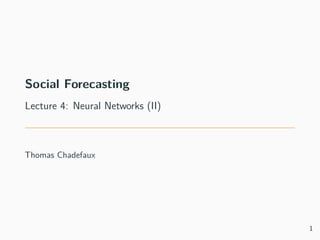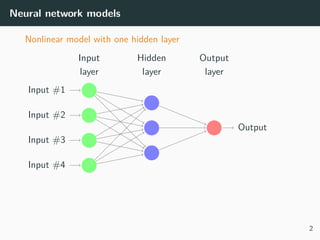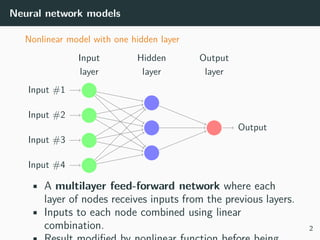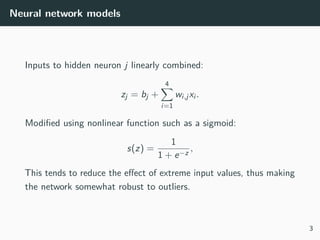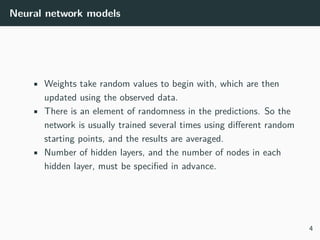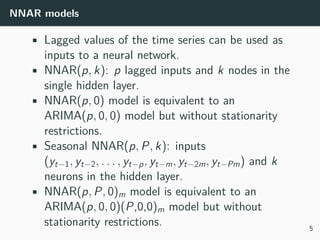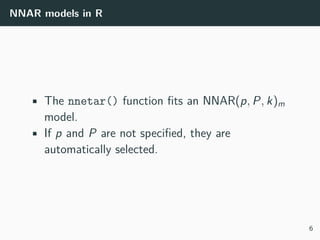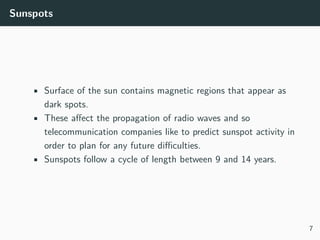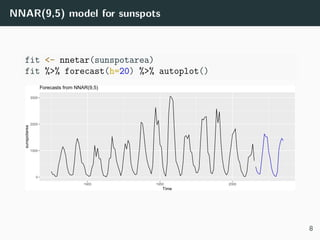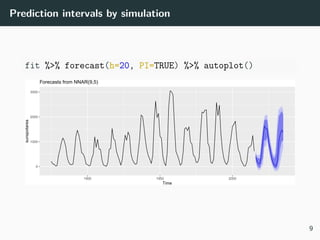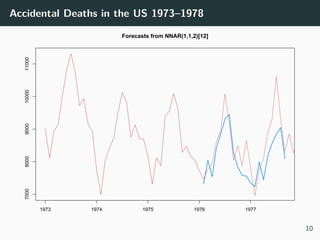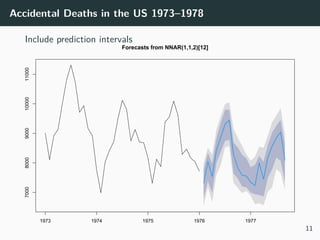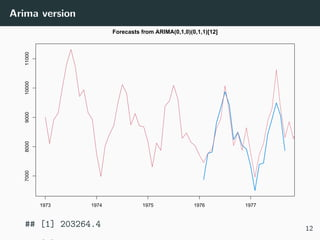1 de 13

### lecture5.pdf

1. Social Forecasting Lecture 4: Neural Networks (II) Thomas Chadefaux 1
2. Neural network models Nonlinear model with one hidden layer Input #1 Input #2 Input #3 Input #4 Output Hidden layer Input layer Output layer 2
3. Neural network models Nonlinear model with one hidden layer Input #1 Input #2 Input #3 Input #4 Output Hidden layer Input layer Output layer • A multilayer feed-forward network where each layer of nodes receives inputs from the previous layers. • Inputs to each node combined using linear combination. 2
4. Neural network models Inputs to hidden neuron j linearly combined: zj = bj + 4 X i=1 wi,jxi . Modified using nonlinear function such as a sigmoid: s(z) = 1 1 + e−z , This tends to reduce the effect of extreme input values, thus making the network somewhat robust to outliers. 3
5. Neural network models • Weights take random values to begin with, which are then updated using the observed data. • There is an element of randomness in the predictions. So the network is usually trained several times using different random starting points, and the results are averaged. • Number of hidden layers, and the number of nodes in each hidden layer, must be specified in advance. 4
6. NNAR models • Lagged values of the time series can be used as inputs to a neural network. • NNAR(p, k): p lagged inputs and k nodes in the single hidden layer. • NNAR(p, 0) model is equivalent to an ARIMA(p, 0, 0) model but without stationarity restrictions. • Seasonal NNAR(p, P, k): inputs (yt−1, yt−2, . . . , yt−p, yt−m, yt−2m, yt−Pm) and k neurons in the hidden layer. • NNAR(p, P, 0)m model is equivalent to an ARIMA(p, 0, 0)(P,0,0)m model but without stationarity restrictions. 5
7. NNAR models in R • The nnetar() function fits an NNAR(p, P, k)m model. • If p and P are not specified, they are automatically selected. 6
8. Sunspots • Surface of the sun contains magnetic regions that appear as dark spots. • These affect the propagation of radio waves and so telecommunication companies like to predict sunspot activity in order to plan for any future difficulties. • Sunspots follow a cycle of length between 9 and 14 years. 7
9. NNAR(9,5) model for sunspots fit <- nnetar(sunspotarea) fit %>% forecast(h=20) %>% autoplot() 0 1000 2000 3000 1900 1950 2000 Time sunspotarea Forecasts from NNAR(9,5) 8
10. Prediction intervals by simulation fit %>% forecast(h=20, PI=TRUE) %>% autoplot() 0 1000 2000 3000 1900 1950 2000 Time sunspotarea Forecasts from NNAR(9,5) 9
11. Accidental Deaths in the US 1973–1978 Forecasts from NNAR(1,1,2) 1973 1974 1975 1976 1977 7000 8000 9000 10000 11000 10
12. Accidental Deaths in the US 1973–1978 Include prediction intervals Forecasts from NNAR(1,1,2) 1973 1974 1975 1976 1977 7000 8000 9000 10000 11000 11
13. Arima version Forecasts from ARIMA(0,1,0)(0,1,1) 1973 1974 1975 1976 1977 7000 8000 9000 10000 11000 ##  203264.4 12